# Control Hardware Electric-Pneumatic Actuation## Control Valve Characteristics

Various types of flow characteristics are available. This tutorial discusses the three main types used in water and steam flow applications: fast opening, linear, and equal percentage flow; how they compare, and how (and why) they should be matched to the application in which they are used.

Flow characteristics

All control valves have an inherent flow characteristic that defines the relationship between ‘valve opening’ and flowrate under constant pressure conditions. Please note that ‘valve opening’ in this context refers to the relative position of the valve plug to its closed position against the valve seat. It does not refer to the orifice pass area. The orifice pass area is sometimes called the ‘valve throat’ and is the narrowest point between the valve plug and seat through which the fluid passes at any time. For any valve, however it is characterised, the relationship between flowrate and orifice pass area is always directly proportional.

Valves of any size or inherent flow characteristic which are subjected to the same volumetric flowrate and differential pressure will have exactly the same orifice pass area. However, different valve characteristics will give different ‘valve openings’ for the same pass area. Comparing linear and equal percentage valves, a linear valve might have a 25% valve opening for a certain pressure drop and flowrate, whilst an equal percentage valve might have a 65% valve opening for exactly the same conditions. The orifice pass areas will be the same.

The physical shape of the plug and seat arrangement, sometimes referred to as the valve ‘trim’, causes the difference in valve opening between these valves. Typical trim shapes for spindle operated globe valves are compared in Figure 6.5.1.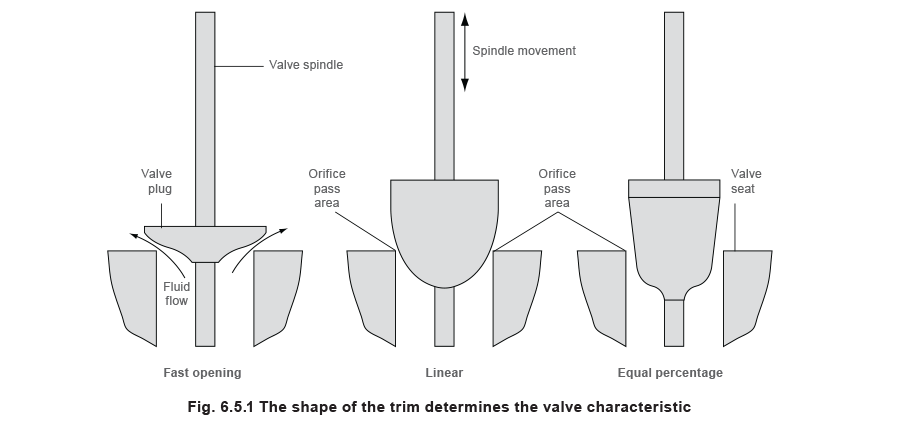In this Module, the term ‘valve lift’ is used to define valve opening, whether the valve is a globe valve (up and down movement of the plug relative to the seat) or a rotary valve (lateral movement of the plug relative to the seat).

Rotary valves (for example, ball and butterfly) each have a basic characteristic curve, but altering the details of the ball or butterfly plug may modify this. The inherent flow characteristics of typical globe valves and rotary valves are compared in Figure 6.5.2.

### Globe valves may be fitted with plugs of differing shapes, each of which has its own inherent flow/opening characteristic. The three main types available are usually designated:

• • Fast opening.
• • Linear.
• • Equal percentage.

Examples of these and their inherent characteristics are shown in Figures 6.5.1 and 6.5.2.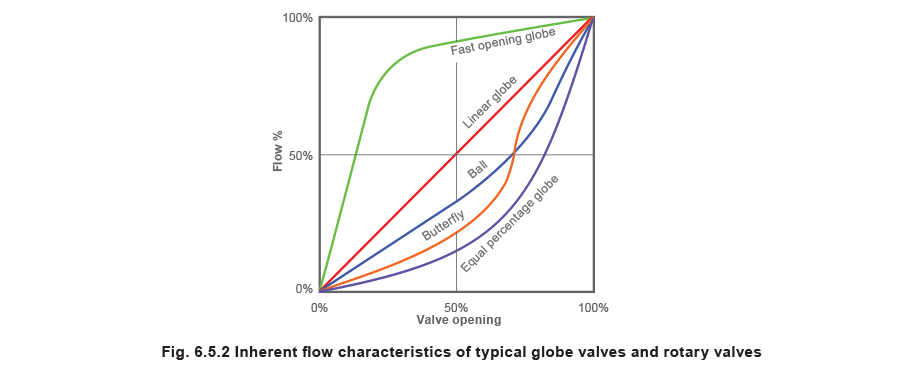Fast opening characteristic

The fast opening characteristic valve plug will give a large change in flowrate for a small valve lift from the closed position. For example, a valve lift of 50% may result in an orifice pass area and flowrate up to 90% of its maximum potential.

A valve using this type of plug is sometimes referred to as having an ‘on/off’ characteristic.

Unlike linear and equal percentage characteristics, the exact shape of the fast opening curve is not defined in standards. Therefore, two valves, one giving a 80% flow for 50% lift, the other 90% flow for 60% lift, may both be regarded as having a fast opening characteristic.

Fast opening valves tend to be electrically or pneumatically actuated and used for ‘on/off’ control.

The self-acting type of control valve tends to have a plug shape similar to the fast opening plug in Figure 6.5.1. The plug position responds to changes in liquid or vapour pressure in the control system. The movement of this type of valve plug can be extremely small relative to small changes in the controlled condition, and consequently the valve has an inherently high rangeability. The valve plug is therefore able to reproduce small changes in flowrate, and should not be regarded as a fast opening control valve.

Linear characteristic

The linear characteristic valve plug is shaped so that the flowrate is directly proportional to the valve lift (H), at a constant differential pressure. A linear valve achieves this by having a linear relationship between the valve lift and the orifice pass area (see Figure 6.5.3).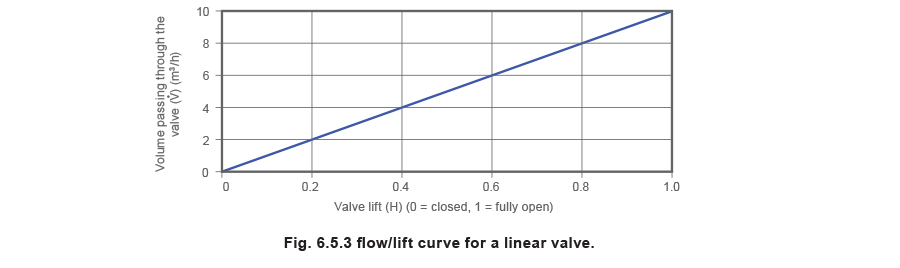For example, at 40% valve lift, a 40% orifice size allows 40% of the full flow to pass.

Equal percentage characteristic (or logarithmic characteristic)

These valves have a valve plug shaped so that each increment in valve lift increases the flowrate by a certain percentage of the previous flow. The relationship between valve lift and orifice size (and therefore flowrate) is not linear but logarithmic, and is expressed mathematically in Equation 6.5.1: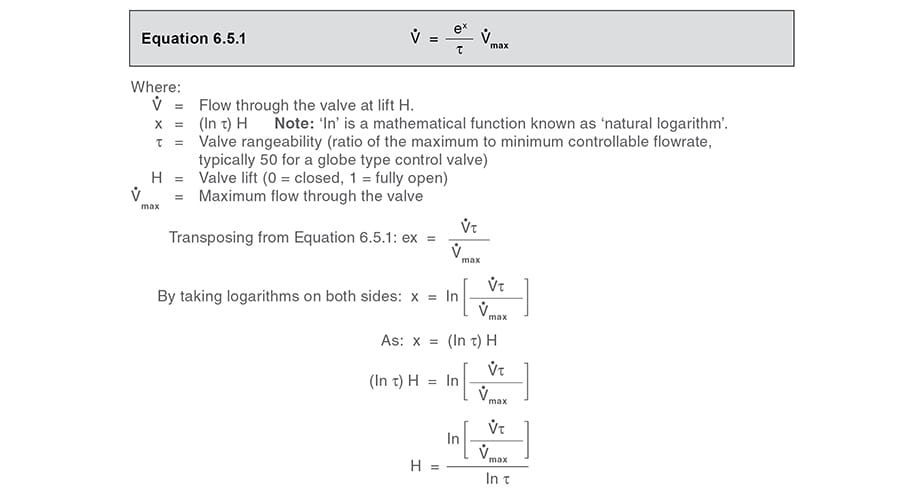Example 6.5.1

The maximum flowrate through a control valve with an equal percentage characteristic is 10 m³/h. If the valve has a turndown of 50:1, and is subjected to a constant differential pressure, by using Equation 6.5.1 what quantity will pass through the valve with lifts of 40%, 50%, and 60% respectively?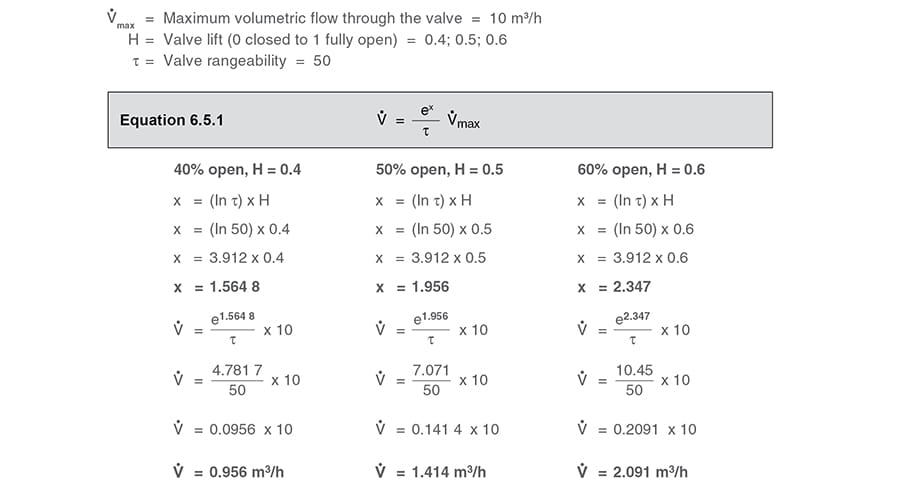The increase in volumetric flowrate through this type of control valve increases by an equal percentage per equal increment of valve movement:

• When the valve is 50% open, it will pass 1.414 m³/h, an increase of 48% over the flow of 0.956 m³/h when the valve is 40% open.
• When the valve is 60% open, it will pass 2.091 m³/h, an increase of 48% over the flow of 1.414 m³/h when the valve is 50% open.

It can be seen that (with a constant differential pressure) for any 10% increase in valve lift, there is a 48% increase in flowrate through the control valve. This will always be the case for an equal percentage valve with rangeability of 50. For interest, if a valve has a rangeability of 100, the incremental increase in flowrate for a 10% change in valve lift is 58%.

Table 6.5.1 shows how the change in flowrate alters across the range of valve lift for the equal percentage valve in Example 6.5.1 with a rangeability of 50 and with a constant differential pressure.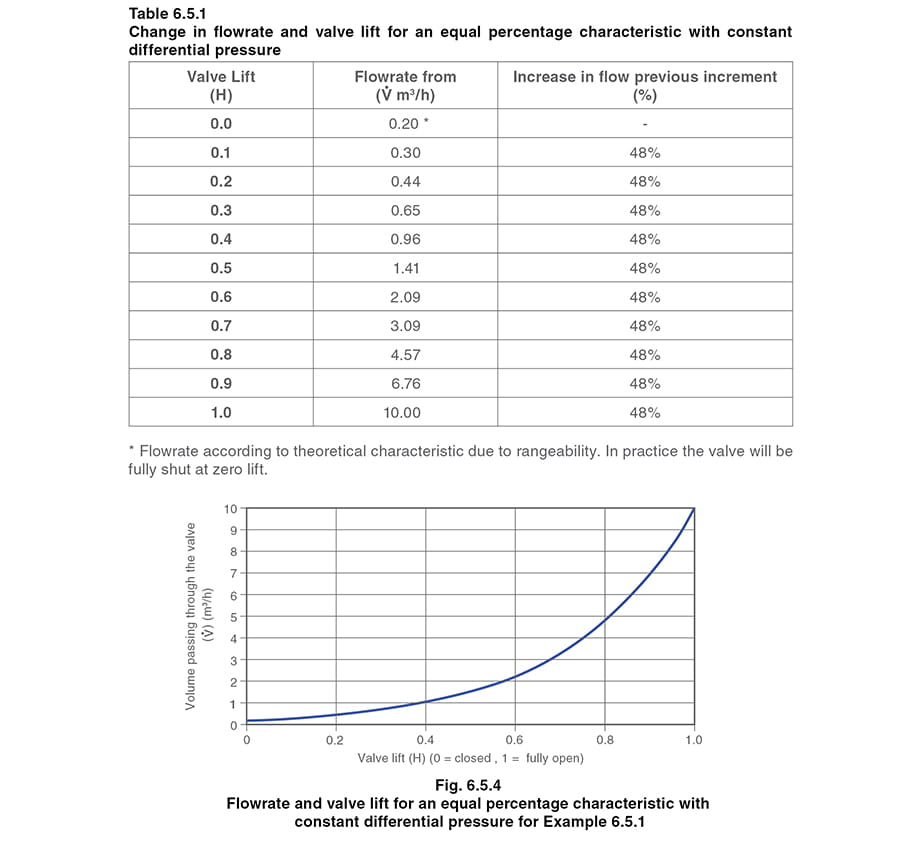A few other inherent valve characteristics are sometimes used, such as parabolic, modified linear or hyperbolic, but the most common types in manufacture are fast opening, linear, and equal percentage.

Matching the valve characteristic to the installation characteristic

Each application will have a unique installation characteristic that relates fluid flow to heat demand. The pressure differential across the valve controlling the flow of the heating fluid may also vary:

• In water systems, the pump characteristic curve means that as flow is reduced, the upstream valve pressure is increased (refer to Example 6.5.2, and Module 6.3).
• In steam temperature control systems, the pressure drop over the control valve is deliberately varied to satisfy the required heat load.

The characteristic of the control valve chosen for an application should result in a direct relationship between valve opening and flow, over as much of the travel of the valve as possible.

This section will consider the various options of valve characteristics for controlling water and steam systems. In general, linear valves are used for water systems whilst steam systems tend to operate better with equal percentage valves.

1. A water circulating heating system with three-port valve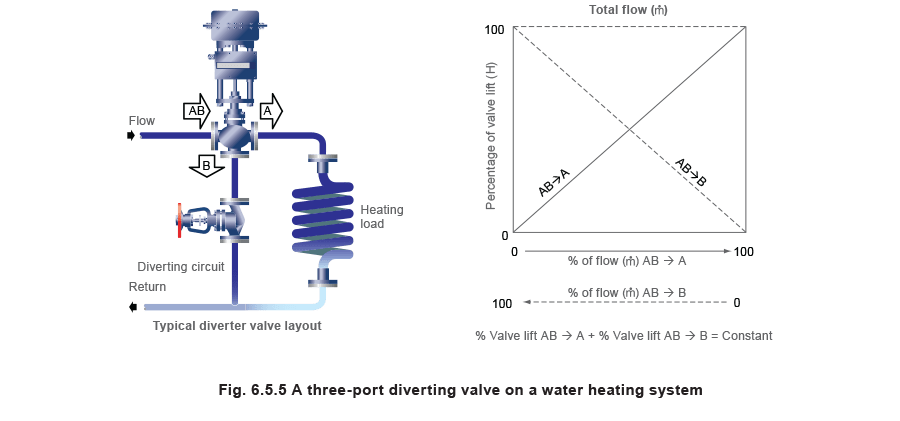In water systems where a constant flowrate of water is mixed or diverted by a three-port valve into a balanced circuit, the pressure loss over the valve is kept as stable as possible to maintain balance in the system.

Conclusion
- The best choice in these applications is usually a valve with a linear characteristic. Because of this, the installed and inherent characteristics are always similar and linear, and there will be limited gain in the control loop.

2. A boiler water level control system – a water system with a two-port valve
valvetwo-port valve

In systems of this type (an example is shown in Figure 6.5.6), where a two-port feedwater control valve varies the flowrate of water, the pressure drop across the control valve will vary with flow. This variation is caused by:

• The pump characteristic. As flowrate is decreased, the differential pressure between the pump and boiler is increased (this phenomenon is discussed in further detail in Module 6.3).
• The frictional resistance of the pipework changes with flowrate. The head lost to friction is proportional to the square of the velocity. (This phenomenon is discussed in further detail in Module 6.3).
• The pressure within the boiler will vary as a function of the steam load, the type of burner control system and its mode of control.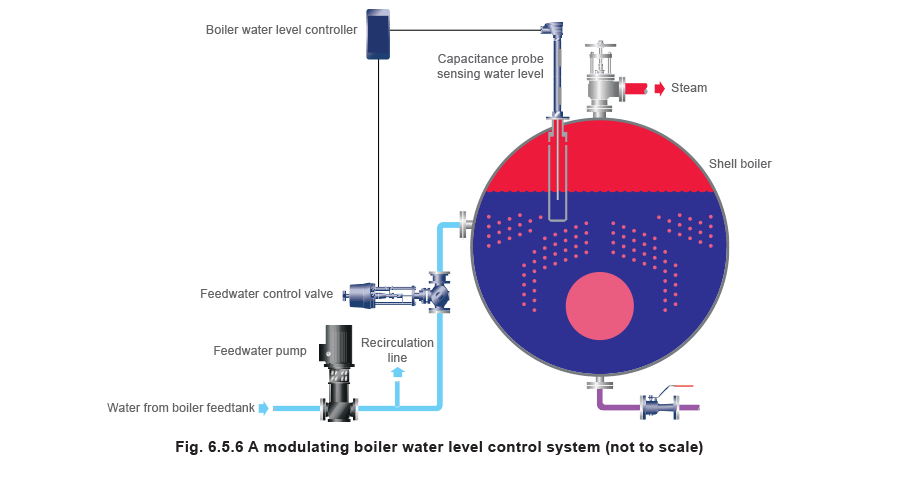Example 6.5.2 Select and size the feedwater valve in Figure 6.5.6

In a simplified example (which assumes a constant boiler pressure and constant friction loss in the pipework), a boiler is rated to produce 10 tonnes of steam per hour. The boiler feedpump performance characteristic is tabulated in Table 6.5.2, along with the resulting differential pressure (ΔP) across the feedwater valve at various flowrates at, and below, the maximum flow requirement of 10 m³/h of feedwater.

Note: The valve ΔP is the difference between the pump discharge pressure and a constant boiler pressure of 10 bar g. Note that the pump discharge pressure will fall as the feedwater flow increases. This means that the water pressure before the feedwater valve also falls with increased flowrate, which will affect the relationship between the pressure drop and the flowrate through the valve.

It can be determined from Table 6.5.2 that the fall in the pump discharge pressure is about 26% from no-load to full-load, but the fall in differential pressure across the feedwater valve is a lot greater at 72%. If the falling differential pressure across the valve is not taken into consideration when sizing the valve, the valve could be undersized.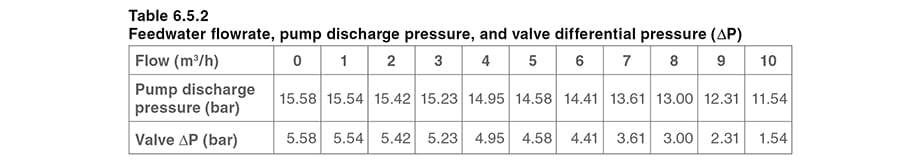As discussed in Modules 6.2 and 6.3, valve capacities are generally measured in terms of Kv. More specifically, Kvs relates to the pass area of the valve when fully open, whilst Kvr relates to the pass area of the valve as required by the application.

Consider if the pass area of a fully open valve with a Kvs of 10 is 100%. If the valve closes so the pass area is 60% of the full-open pass area, the Kvr is also 60% of 10 = 6. This applies regardless of the inherent valve characteristic. The flowrate through the valve at each opening will depend upon the differential pressure at the time.

Using the data in Table 6.5.2, the required valve capacity, Kvr, can be calculated for each incremental flowrate and valve differential pressure, by using Equation 6.5.2, which is derived from Equation 6.3.2.The Kvr can be thought of as being the actual valve capacity required by the installation and, if plotted against the required flowrate, the resulting graph can be referred to as the ‘installation curve’.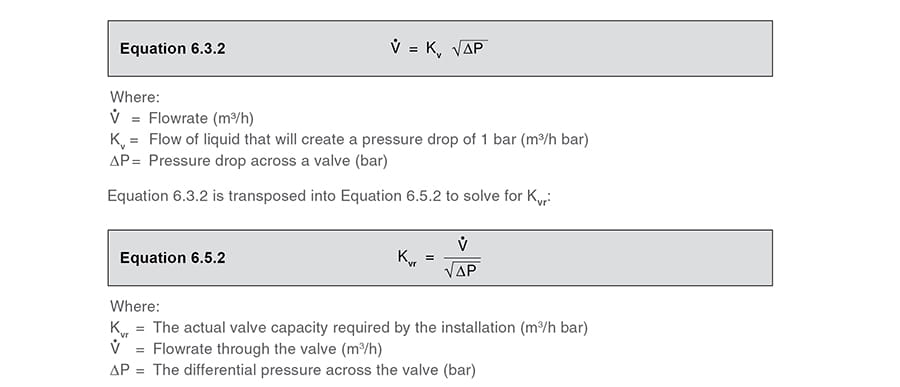At the full-load condition, from Table 6.5.2:

Required flow through the valve = 10 m³/ h

ΔP across the valve = 1.54 bar

From Equation 6.5.2:

ΔP across the valve = 1.54 bar

From Equation 6.5.2:barTaking the valve flowrate and valve ΔP from Table 6.5.2, a Kvr for each increment can be determined from Equation 6.5.2; and these are tabulated in Table 6.5.3.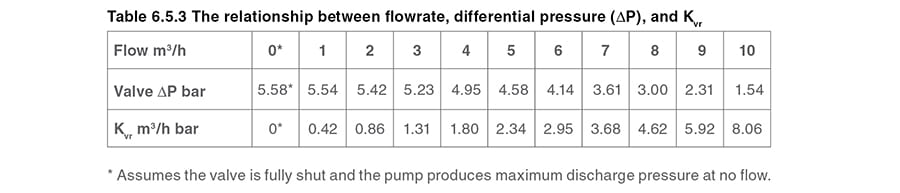Constructing the installation curve

The Kvr of 8.06 satisfies the maximum flow condition of 10 m3/h for this example.

The installation curve could be constructed by comparing flowrate to Kvr, but it is usually more convenient to view the installation curve in percentage terms. This simply means the percentage of Kvr to Kvs, or in other words, the percentage of actual pass area relative to the full open pass area.

For this example: The installation curve is constructed, by taking the ratio of Kvr at any load relative to the Kvs of 8.06. A valve with a Kvs of 8.06 would be ‘perfectly sized’, and would describe the installation curve, as tabulated in Table 6.5.4, and drawn in Figure 6.5.7. This installation curve can be thought of as the valve capacity of a perfectly sized valve for this example.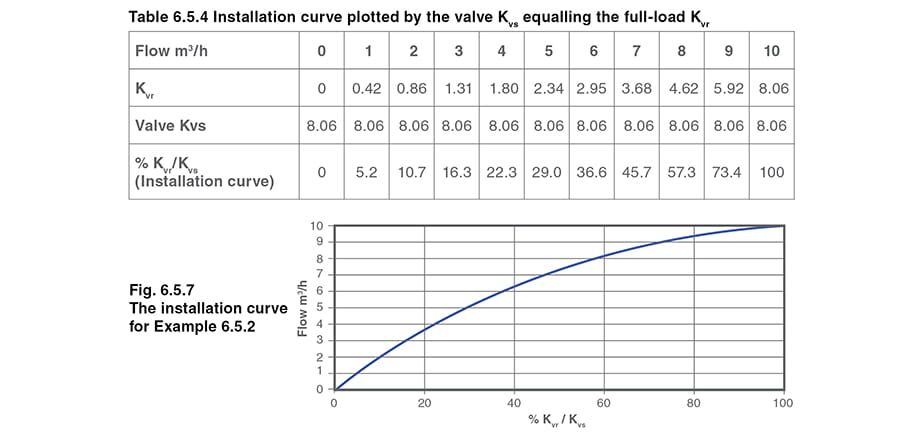It can be seen that, as the valve is ‘perfectly sized’ for this installation, the maximum flowrate is satisfied when the valve is fully open.

However, it is unlikely and undesirable to select a perfectly sized valve. In practice, the selected valve would usually be at least one size larger, and therefore have a Kvs larger than the installation Kvr.

As a valve with a Kvs of 8.06 is not commercially available, the next larger standard valve would have a Kvs of 10 with nominal DN25 connections.

It is interesting to compare linear and equal percentage valves having a Kvs of 10 against the installation curve for this example.

Consider a valve with a linear inherent characteristic

A valve with a linear characteristic means that the relationship between valve lift and orifice pass area is linear. Therefore, both the pass area and valve lift at any flow condition is simply the Kvr expressed as a proportion of the valve Kvs. For example: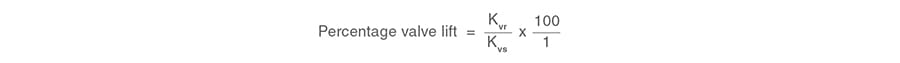It can be seen from Table 6.5.4, that at the maximum flowrate of 10 m³/h, the Kvr is 8.06. If the linear valve has a Kvs of 10, for the valve to satisfy the required maximum flowrate, the valve will lift: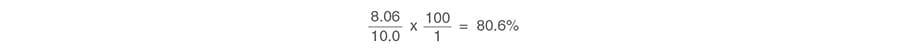Using the same routine, the orifice size and valve lift required at various flowrates may be determined for the linear valve, as shown in Table 6.5.5.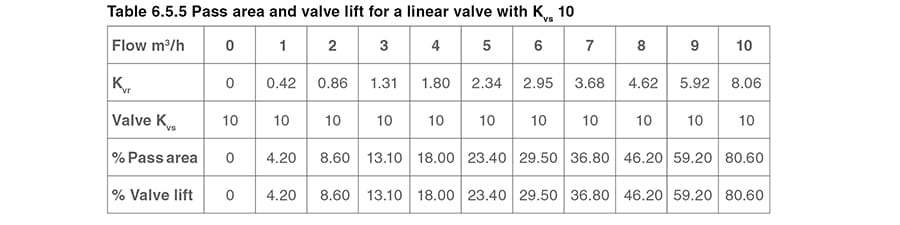An equal percentage valve will require exactly the same pass area to satisfy the same maximum flowrate, but its lift will be different to that of the linear valve.

Consider a valve with an equal percentage inherent characteristic
Given a valve rangeability of 50:1, τ  = 50, the lift (H) may be determined using Equation 6.5.1:Percentage valve lift is denoted by Equation 6.5.3.As the volumetric flowrate through any valve is proportional to the orifice pass area, Equation 6.5.3 can be modified to give the equal percentage valve lift in terms of pass area and therefore Kv.

This is shown by Equation 6.5.4.As already calculated, the Kvr at the maximum flowrate of 10 m³/h is 8.06, and the Kvs of the DN25 valve is 10. By using Equation 6.5.4 the required valve lift at full-load is therefore:
therefore:Using the same routine, the valve lift required at various flowrates can be determined from Equation 6.5.4 and is shown in Table 6.5.6.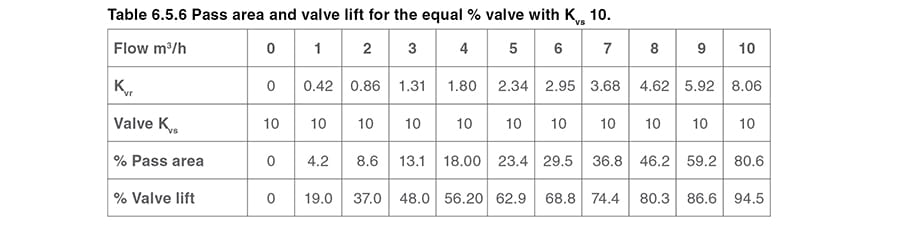Comparing the linear and equal percentage valves for this application

The resulting application curve and valve curves for the application in Example 6.5.2 for both the linear and equal percentage inherent valve characteristics are shown in Figure 6.5.8.

Note that the equal percentage valve has a significantly higher lift than the linear valve to achieve the same flowrate. It is also interesting to see that, although each of these valves has a Kvs larger than a ‘perfectly sized valve’ (which would produce the installation curve), the equal percentage valve gives a significantly higher lift than the installation curve. In comparison, the linear valve always has a lower lift than the installation curve.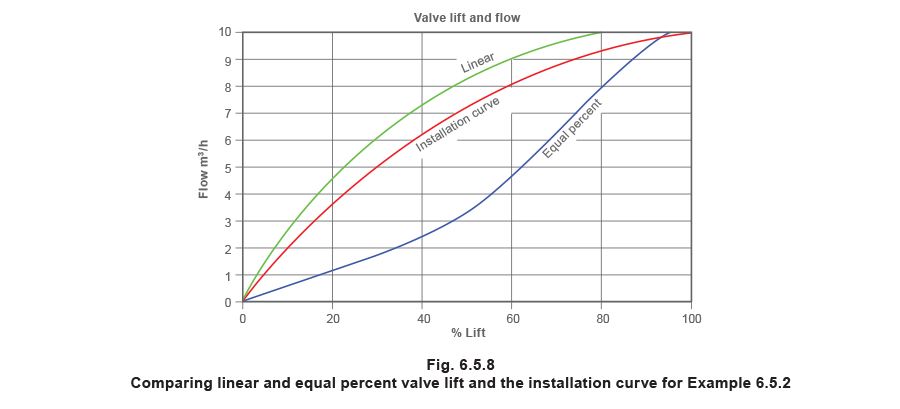The rounded nature of the curve for the linear valve is due to the differential pressure falling across the valve as the flow increases. If the pump pressure had remained constant across the whole range of flowrates, the installation curve and the curve for the linear valve would both have been straight lines.

By observing the curve for the equal percentage valve, it can be seen that, although a linear relationship is not achieved throughout its whole travel, it is above 50% of the flowrate.

The equal percentage valve offers an advantage over the linear valve at low flowrates. Consider, at a 10% flowrate of 1 m³/h, the linear valve only lifts roughly 4%, whereas the equal percentage valve lifts roughly 20%. Although the orifice pass area of both valves will be exactly the same, the shape of the equal percentage valve plug means that it operates further away from its seat, reducing the risk of impact damage between the valve plug and seat due to quick reductions in load at low flowrates.

An oversized equal percentage valve will still give good control over its full range, whereas an oversized linear valve might perform less effectively by causing fast changes in flowrate for small changes in lift.

Conclusion - In most applications, an equal percentage valve will provide good results, and is very tolerant of over-sizing. It will offer a more constant gain as the load changes, helping to provide a more stable control loop at all times. However, it can be observed from Figure 6.5.8, that if the linear valve is properly sized, it will perform perfectly well in this type of water application.

3. Temperature control of a steam application with a two-port valve

In heat exchangers, which use steam as the primary heating agent, temperature control is achieved by varying the flow of steam through a two-port control valve to match the rate at which steam condenses on the heating surfaces. This varying steam flow varies the pressure (and hence temperature) of the steam in the heat exchanger and thus the rate of heat transfer.

Example 6.5.3

In a particular steam-to-water heat exchange process, it is proposed that:

• Water is heated from 10°C to a constant 60°C.
• The water flowrate varies between 0 and 10 L/s (kg/s).
• At full-load, steam is required at 4 bar a in the heat exchanger coils.
• The overall heat transfer coefficient (U) is 1 500 W/m2°C at full-load, and reduces by 4% for every 10% drop in secondary water flowrate.

Using this data, and by applying the correct equations, the following properties can be determined:

• The heat transfer area to satisfy the maximum load. Not until this is established can the following be found:
• The steam temperature at various heat loads.
• The steam pressure at various heat loads.

• Find the heat load.

Heat load is determined from Equation 2.6.5: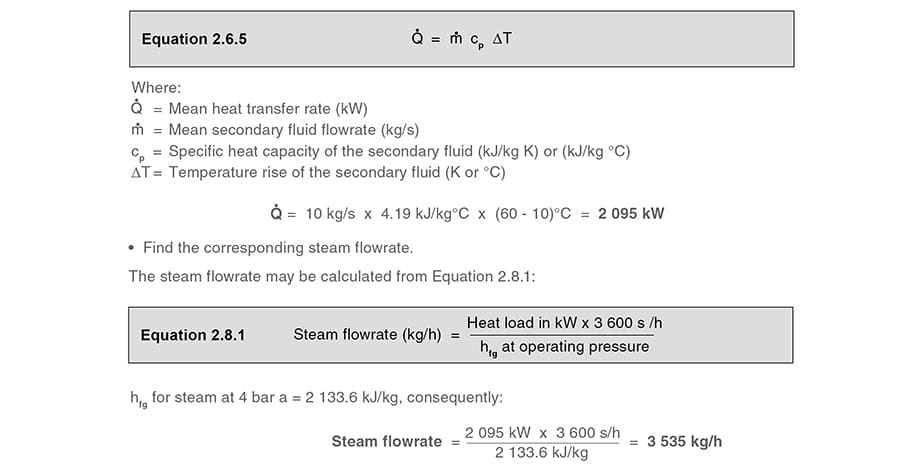• Find the heat transfer area required to satisfy the maximum load.

The heat transfer area (A) can be determined from Equation 2.5.3:At this stage, ΔTLM is unknown, but can be calculated from the primary steam and secondary water temperatures, using Equation 2.5.5.

• Find the log mean temperature difference.

ΔTLM may be determined from Equation 2.5.5: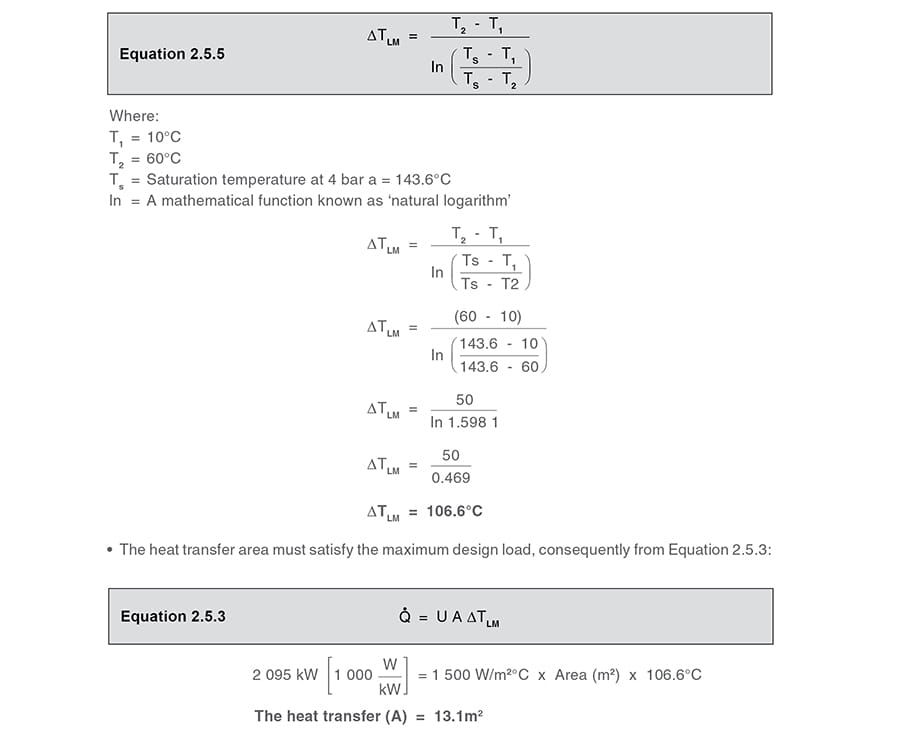Find the conditions at other heat loads at a 10% reduced water flowrate:

• Find the heat load.

If the water flowrate falls by 10% to 9 kg/s, the heat load reduces to:

Q̇ = 9 kg/s x (60 – 10°C) x 4.19 kJ / kg °C = 1 885.5 kW

The initial ‘U’ value of 1 500 W/m2 °C is reduced by 4%, so the temperature required in the steam space may be calculated from Equation 2.5.3: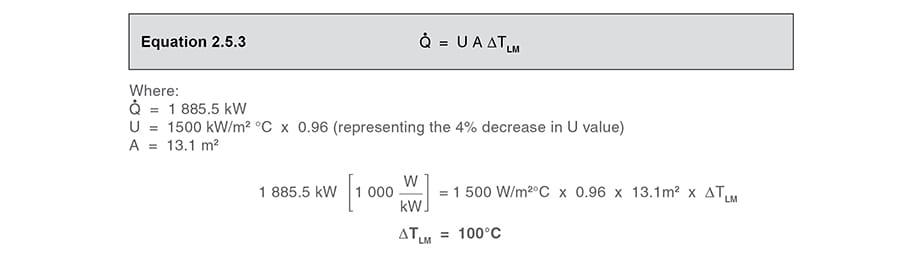• Find the steam temperature at this reduced load.

If ΔTLM = 100°C, and T1, T2 are already known, then Ts may be determined from Equation 2.5.5: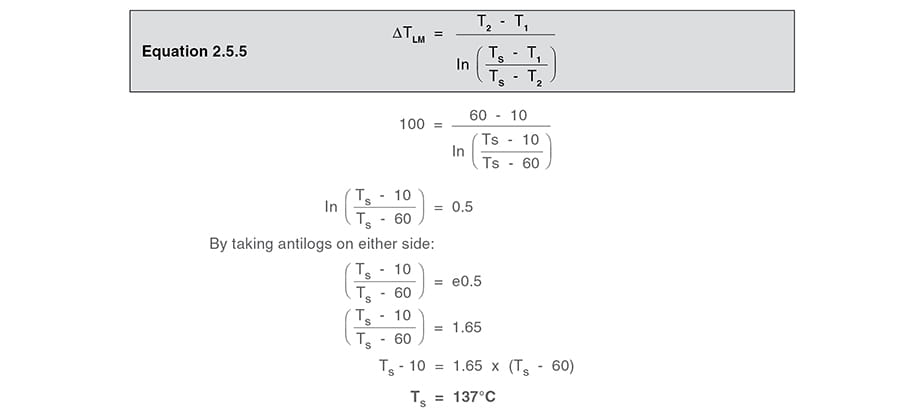• Find the steam flowrate.

The saturated steam pressure for 137°C is 3.32 bar a (from the Spirax Sarco steam tables).

At 3.32 bar a, hfg = 2 153.5 kJ/kg, consequently from Equation 2.8.1:Using this routine, a set of values may be determined over the operating range of the heat exchanger, as shown in Table 6.5.7.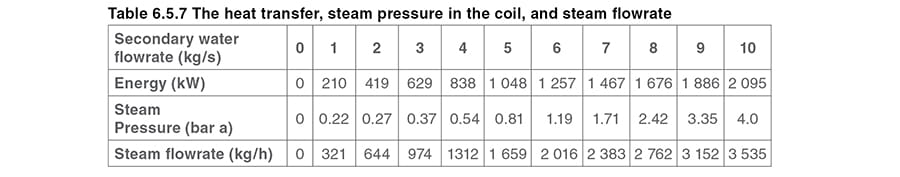If the steam pressure supplying the control valve is given as 5.0 bar a, and using the steam pressure and steam flowrate information from Table 6.5.7; the Kvr can be calculated from Equation 6.5.6, which is derived from the steam flow formula, Equation 3.21.2.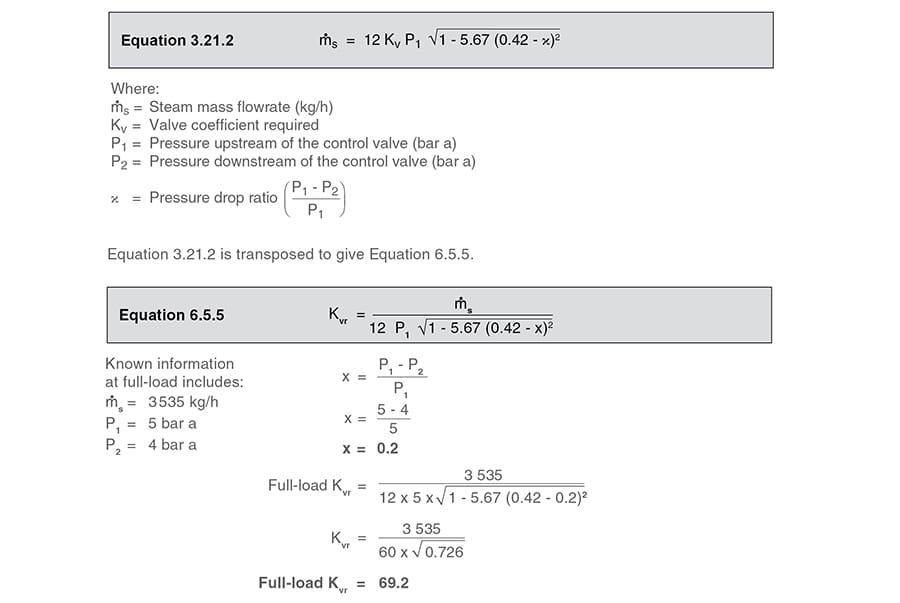Using this routine, the Kvr for each increment of flow can be determined, as shown in Table 6.5.8.

The installation curve can also be defined by considering the Kvr at all loads against the ‘perfectly sized’ Kvs of 69.2.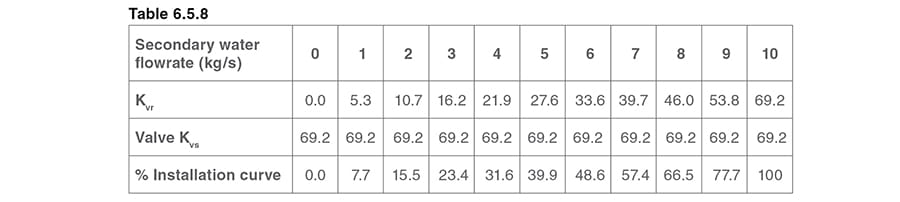The Kvr of 69.2 satisfies the maximum secondary flow of 10 kg / s.

In the same way as in Example 6.5.2, the installation curve is described by taking the ratio of Kvr at any load relative to a Kvs of 69.2.

Such a valve would be ‘perfectly sized’ for the example, and would describe the installation curve, as tabulated in Table 6.5.8, and drawn in Figure 6.5.9.

The installation curve can be thought of as the valve capacity of a valve perfectly sized to match the application requirement.

It can be seen that, as the valve with a Kvs of 69.2 is ‘perfectly sized’ for this application, the maximum flowrate is satisfied when the valve is fully open.

However, as in the water valve sizing Example 6.5.2, it is undesirable to select a perfectly sized valve. In practice, it would always be the case that the selected valve would be at least one size larger than that required, and therefore have a Kvs larger than the application Kvr.

A valve with a Kvs of 69.2 is not commercially available, and the next larger standard valve has a Kvs of 100 with nominal DN80 connections.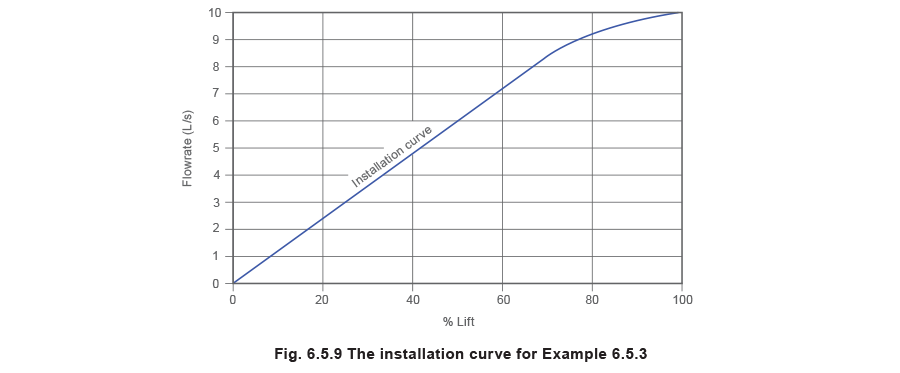It is interesting to compare linear and equal percentage valves having a Kvs of 100 against the installation curve for this example.

Consider a valve with a linear inherent characteristic

A valve with a linear characteristic means that the relationship between valve lift and orifice pass area is linear. Therefore, both the pass area and valve lift at any flow condition is simply the Kvr expressed as a proportion of the valve Kvs. For example.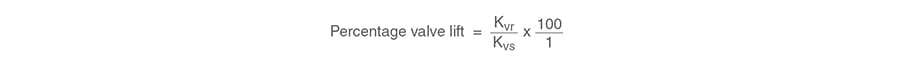At the maximum water flowrate of 10 kg/s, the steam valve Kvr is 69.2. The Kvs of the selected valve is 100, consequently the lift is: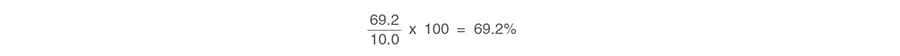Using the same procedure, the linear valve lifts can be determined for a range of flows, and are tabulated in Table 6.5.9.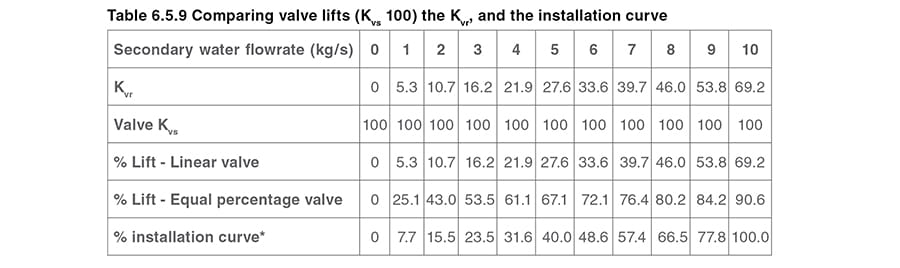Consider a valve with an equal percentage inherent characteristic

An equal percentage valve will require exactly the same pass area to satisfy the same maximum flowrate, but its lift will be different to that of the linear valve.

Given that the valve turndown ratio, τ = 50, the lift (H) may be determined using Equation 6.5.4.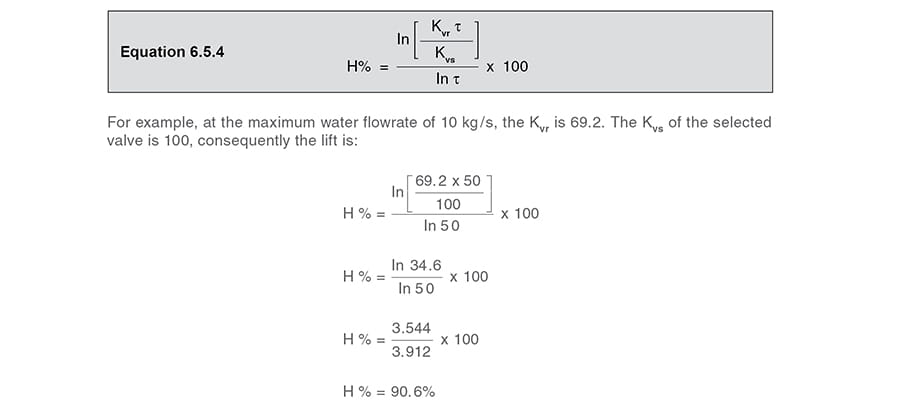Using the same procedure, the percentage valve lift can be determined from Equation 6.5.4 for a range of flows for this installation.

The corresponding lifts for linear and equal percentage valves are shown in Table 6.5.9 along with the installation curve.

As in Example 6.5.2, the equal percentage valve requires a much higher lift than the linear valve to achieve the same flowrate. The results are graphed in Figure 6.5.10.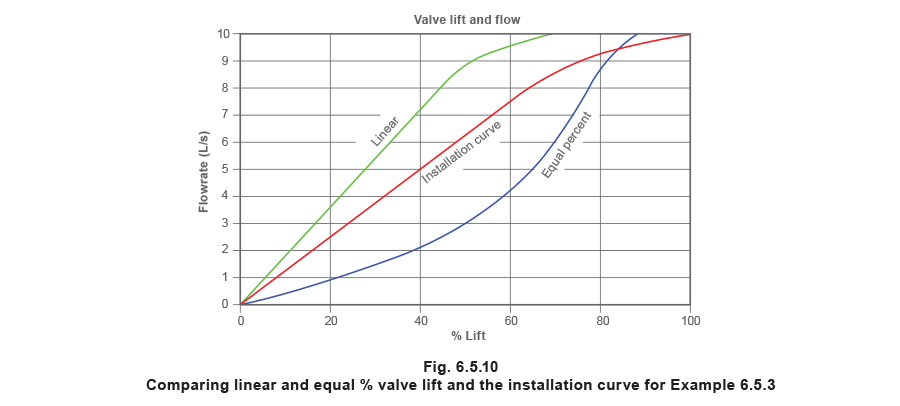There is a sudden change in the shape of the graphs at roughly 90% of the load; this is due to the effect of critical pressure drop across the control valve which occurs at this point.

Above 86% load in this example, it can be shown that the steam pressure in the heat exchanger is above 2.9 bar a which, with 5 bar a feeding the control valve, is the critical pressure value. (For more information on critical pressure, refer to Module 6.4, Control valve sizing for steam).

It is generally agreed that control valves find it difficult to control below 10% of their range, and in practice, it is usual for them to operate between 20% and 80% of their range.

The graphs in Figure 6.5.10 refer to linear and equal percentage valves having a Kvs of 100, which are the next larger standard valves with suitable capacity above the application curve (the required Kvr of 69.2), and would normally be chosen for this particular example.

The effect of a control valve which is larger than necessary

It is worth while considering what effect the next larger of the linear or equal percentage valves would have had if selected. To accommodate the same steam loads, each of these valves would have had lower lifts than those observed in Figure 6.5.10.

The next larger standard valves have a Kvs of 160. It is worth noting how these valves would perform should they have been selected, and as shown in Table 6.5.10 and Figure 6.5.11.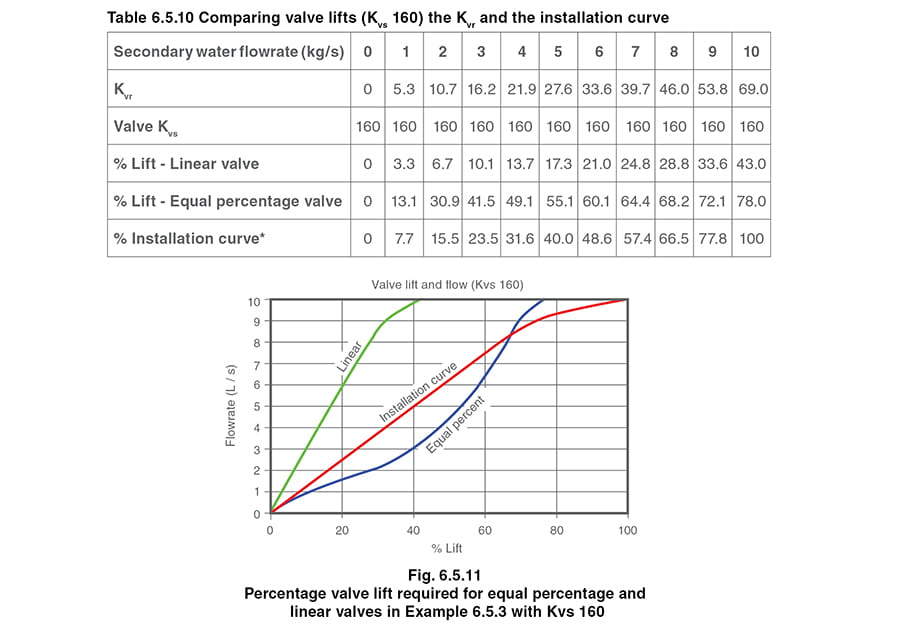It can be seen from Figure 6.5.11 that both valve curves have moved to the left when compared to the smaller (properly sized) valves in Figure 6.5.10, whilst the installation curve remains static.

The change for the linear valve is quite dramatic; it can be seen that, at 30% load, the valve is only 10% open. Even at 85% load, the valve is only 30% open. It may also be observed that the change in flowrate is large for a relatively small change in the lift. This effectively means that the valve is operating as a fast acting valve for up to 90% of its range. This is not the best type of inherent characteristic for this type of steam installation, as it is usually better for changes in steam flow to occur fairly slowly.

Although the equal percentage valve curve has moved position, it is still to the right of the installation curve and able to provide good control. The lower part of its curve is relatively shallow, offering slower opening during its initial travel, and is better for controlling steam flow than the linear valve in this case.

Circumstances that can lead to over-sizing include:

• The application data is approximate, consequently an additional ‘safety factor’ is included.
• Sizing routines that include operational ‘factors’ such as an over-zealous allowance for fouling.
• The calculated Kvr is only slightly higher than the Kvs of a standard valve, and the next larger size has to be selected.

There are also situations where:

• The available pressure drop over the control valve at full-load is low.

For example, if the steam supply pressure is 4.5 bar a and the steam pressure required in the heat exchanger at full-load is 4 bar a, this only gives an 11% pressure drop at full-load.

• The minimum load is a lot less than the maximum load

A linear valve characteristic would mean that the valve plug operates close to the seat, with the possibility of damage.

In these common circumstances, the equal percentage valve characteristic will provide a much more flexible and practical solution.

This is why most control valve manufacturers will recommend an equal percentage characteristic for two-port control valves, especially when used on compressible fluids such as steam.

Please note: Given the opportunity, it is better to size steam valves with as high a pressure drop as possible at maximum load; even with critical pressure drop occurring across the control valve if the conditions allow. This helps to reduce the size and cost of the control valve, gives a more linear installation curve, and offers an opportunity to select a linear valve.

However, conditions may not allow this. The valve can only be sized on the application conditions. For example, should the heat exchanger working pressure be 4.5 bar a, and the maximum available steam pressure is only 5 bar a, the valve can only be sized on a 10% pressure drop ([5 – 4.5]/5). In this situation, sizing the valve on critical pressure drop would have reduced the size of the control valve and starved the heat exchanger of steam.

If it were impossible to increase the steam supply pressure, a solution would be to install a heat exchanger that operates at a lower operating pressure. In this way, the pressure drop would increase across the control valve. This could result in a smaller valve but also a larger heat exchanger, because the heat exchanger operating temperature is now lower.

Another set of advantages accrues from larger heat exchangers operating at lower steam pressures:

• There is less propensity for scaling and fouling on the heating surfaces.
• There is less flash steam produced in the condensate system.
• There is less backpressure in the condensate system.

A balance has to be made between the cost of the control valve and heat exchanger, the ability of the valve to control properly, and the effects on the rest of the system as seen above. On steam systems, equal percentage valves will usually be a better choice than linear valves, because if low pressure drops occur, they will have less of an affect on their performance over the complete range of valve movement.